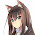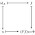Sunday, October 26, 2014

Haskell's core language is very small, and most Haskell code desugars to either:

• lambdas / function application,
• algebraic data types / case expressions,
• recursive `let` bindings,
• type classes and specialization, or:
• Foreign function calls

Once you understand those concepts you have a foundation for understanding everything else within the language. As a result, the language feels very small and consistent.

I'll illustrate how many higher-level features desugar to the same set of lower-level primitives.

if

`if` is equivalent to a `case` statement:

``````if b then e1 else e2

-- ... is equivalent to:
case b of
True  -> e1
False -> e2``````

This works because `Bool`s are defined within the language:

``data Bool = False | True``

Multi-argument lambdas

Lambdas of multiple arguments are equivalent to nested lambdas of single arguments:

``````\x y z -> e

-- ... is equivalent to:
\x -> \y -> \z -> e``````

Functions

Functions are equivalent to lambdas:

``````f x y z = e

-- ... is equivalent to:
f = \x y z -> e

-- ... which in turn desugars to:
f = \x -> \y -> \z -> e``````

As a result, all functions of multiple arguments are really just nested functions of one argument each. This trick is known as "currying".

Infix functions

You can write functions of at least two arguments in infix form using backticks:

``````x `f` y

-- ... desugars to:
f x y``````

Operators

Operators are just infix functions of two arguments that don't need backticks. You can write them in prefix form by surrounding them with parentheses:

``````x + y

-- ... desugars to:
(+) x y``````

The compiler distinguishes operators from functions by reserving a special set of punctuation characters exclusively for operators.

Operator parameters

The parentheses trick for operators works in other contexts, too. You can bind parameters using operator-like names if you surround them with parentheses:

``````let f (%) x y = x % y
in  f (*) 1 2

-- ... desugars to:
(\(%) x y -> x % y) (*) 1 2

-- ... reduces to:
1 * 2``````

Operator sections

You can partially apply operators to just one argument using a section:

``````(1 +)

-- desugars to:
\x -> 1 + x``````

This works the other way, too:

``````(+ 1)

-- desugars to:
\x -> x + 1``````

This also works with infix functions surrounded by backticks:

``````(`f` 1)

-- desugars to:
\x -> x `f` 1

-- desugars to:
\x -> f x 1``````

Pattern matching

Pattern matching on constructors desugars to case statements:

``````f (Left  l) = eL
f (Right r) = eR

-- ... desugars to:
f x = case x of
Left  l -> eL
Right r -> eR``````

Pattern matching on numeric or string literals desugars to equality tests:

``````f 0 = e0
f _ = e1

-- ... desugars to:
f x = if x == 0 then e0 else e1

-- ... desugars to:
f x = case x == 0 of
True  -> e0
False -> e1``````

Non-recursive let / where

Non-recursive `let`s are equivalent to lambdas:

``````let x = y in z

-- ... is equivalent to:
(\x -> z) y``````

Same thing for `where`, which is identical in purpose to `let`:

``````z where x = y

-- ... is equivalent to:
(\x -> z) y``````
Actually, that's not quite true, because of let generalization, but it's close to the truth.

Recursive `let` / `where` cannot be desugared like this and should be treated as a primitive.

Top-level functions

Multiple top-level functions can be thought of as one big recursive `let` binding:

``````f0 x0 = e0

f1 x1 = e1

main = e2

-- ... is equivalent to:
main = let f0 x0 = e0
f1 x1 = e1
in  e2``````

In practice, Haskell does not desugar them like this, but it's a useful mental model.

Imports

Importing modules just adds more top-level functions. Importing modules has no side effects (unlike some languages), unless you use Template Haskell.

Type-classes

Type classes desugar to records of functions under the hood where the compiler implicitly threads the records throughout the code for you.

``````class Monoid m where
mappend :: m -> m -> m
mempty :: m

instance Monoid Int where
mappend = (+)
mempty  = 0

f :: Monoid m => m -> m
f x = mappend x x

-- ... desugars to:
data Monoid m = Monoid
{ mappend :: m -> m -> m
, mempty  :: m
}

intMonoid :: Monoid Int
intMonoid = Monoid
{ mappend = (+)
, mempty  = 0
}

f :: Monoid m -> m -> m
f (Monoid p z) x = p x x``````

... and specializing a function to a particular type class just supplies the function with the appropriate record:

``````g :: Int -> Int
g = f

-- ... desugars to:
g = f intMonoid``````

Two-line `do` notation

A two-line `do` block desugars to the infix `(>>=)` operator:

``````do x <- m
e

-- ... desugars to:
m >>= (\x ->
e )``````

One-line `do` notation

For a one-line `do` block, you can just remove the `do`:

``````main = do putStrLn "Hello, world!"

-- ... desugars to:
main = putStrLn "Hello, world!"``````

Multi-line `do` notation

`do` notation of more than two lines is equivalent to multiple nested `do`s:

``````do x <- mx
y <- my
z

-- ... is equivalent to:
do x <- mx
do y <- my
z

-- ... desugars to:
mx >>= (\x ->
my >>= (\y ->
z ))``````

`let` in `do` notation

Non-recursive `let` in a `do` block desugars to a lambda:

``````do let x = y
z

-- ... desugars to:
(\x -> z) y``````

`ghci`

The `ghci` interactive REPL is analogous to one big `do` block (with lots and lots of caveats):

``````\$ ghci
>>> str <- getLine
>>> let str' = str ++ "!"
>>> putStrLn str'

-- ... is equivalent to the following Haskell program:
main = do
str <- getLine
let str' = str ++ "!"
putStrLn str'

-- ... desugars to:
main = do
str <- getLine
do let str' = str ++ "!"
putStrLn str'

-- ... desugars to:
main =
getLine >>= (\str ->
do let str' = str ++ "!"
putStrLn str' )

-- ... desugars to:
main =
getLine >>= (\str ->
(\str' -> putStrLn str') (str ++ "!") )

-- ... reduces to:
main =
getLine >>= (\str ->
putStrLn (str ++ "!") )``````

List comprehensions

List comprehensions are equivalent to `do` notation:

``````[ (x, y) | x <- mx, y <- my ]

-- ... is equivalent to:

do x <- mx
y <- my
return (x, y)

-- ... desugars to:
mx >>= (\x -> my >>= \y -> return (x, y))

-- ... specialization to lists:
concatMap (\x -> concatMap (\y -> [(x, y)]) my) mx``````

The real desugared code is actually more efficient, but still equivalent.

The `MonadComprehensions` language extension generalizes list comprehension syntax to work with any `Monad`. For example, you can write an `IO` comprehension:

``````>>> :set -XMonadComprehensions
>>> [ (str1, str2) | str1 <- getLine, str2 <- getLine ]
Line1<Enter>
Line2<Enter>
("Line1", "Line2")``````

Numeric literals

Integer literals are polymorphic by default and desugar to a call to `fromIntegral` on a concrete `Integer`:

``````1 :: Num a => a

-- desugars to:
fromInteger (1 :: Integer)``````

Floating point literals behave the same way, except they desugar to `fromRational`:

``````1.2 :: Fractional a => a

-- desugars to:
fromRational (1.2 :: Rational)``````

IO

You can think of `IO` and all foreign function calls as analogous to building up a syntax tree describing all planned side effects:

``````main = do
str <- getLine
putStrLn str
return 1

-- ... is analogous to:
data IO r
= PutStrLn String (IO r)
| GetLine (String -> IO r)
| Return r

(PutStrLn str io) >>= f = PutStrLn str (io >>= f)
(GetLine k      ) >>= f = GetLine (\str -> k str >>= f)
Return r          >>= f = f r

main = do
str <- getLine
putStrLn str
return 1

-- ... desugars and reduces to:
main =
GetLine (\str ->
PutStrLn str (
Return 1 ))``````

This mental model is actually very different from how `IO` is implemented under the hood, but it works well for building an initial intuition for `IO`.

For example, one intuition you can immediately draw from this mental model is that order of evaluation in Haskell has no effect on order of `IO` effects, since evaluating the syntax tree does not actually interpret or run the tree. The only way you can actually animate the syntax tree is to define it to be equal to `main`.

Conclusion

I haven't covered everything, but hopefully that gives some idea of how Haskell translates higher level abstractions into lower-level abstractions. By keeping the core language small, Haskell can ensure that language features play nicely with each other.

Note that Haskell also has a rich ecosystem of experimental extensions, too. Many of these are experimental because each new extension must be vetted to understand how it interacts with existing language features.

1.“Non-recursive lets are equivalent to lambdas:” This is true superficially, but intermediate languages (like Core) still have non-recursive lets, as they have to be treated quite differently by an optimizing compiler: Not much is known about a lambda-abstracted variable, but a let-bound value is known completely.

1.You're right. I added a caveat mentioning that this is not completely true because of let generalization.

2.Interesting! This is pretty close to 'free theorem equivalence' - I noticed your Morte could likely encode a free groupoid. These should cover a lot of it, and be sure to read the comments in each of the first two:
https://golem.ph.utexas.edu/category/2008/01/mark_weber_on_nerves_of_catego.html
http://arxiv.org/pdf/1101.3064.pdf
http://www.frontiersinai.com/turingfiles/March/03Tessonconservatives-LATA2012.pdf

3.if b then e1 else e2

-- ... is equivalent to:
case b of
True -> e1
False -> e2

4.Thanks to deniok@lj:

Prelude> (\True y -> ()) False `seq` 5
5
Prelude> (\True -> \y -> ()) False `seq` 5
*** Exception: :3:2-18: Non-exhaustive patterns in lambda

1.s/deniok/deni-ok/

5.Desugaring the do notation is not as simple as shown here because of `fail`. See https://wiki.haskell.org/MonadFail_Proposal for details. Here is the relevant excerpt:

"
Currently, the <- symbol is unconditionally desugared as follows:

do pat <- computation

becomes

let f pat = more
f _ = fail "..."
in computation >>= f
"